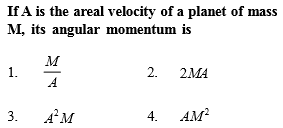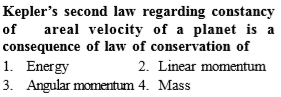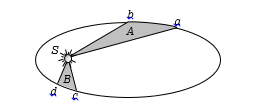You’ve reached the end of your free Videos limit.
#3 | Kepler's 3rd Law
(Physics) > Gravitation
Related Practice Questions :Complete Question Bank + Test Series
Complete Question Bank

Difficulty Level:Complete Question Bank + Test Series
Complete Question Bank

Difficulty Level:

The distance of neptune and saturn from sun are nearly ${10}^{13}$ and ${10}^{12}$ meters respectively. Assuming that they move in circular orbits, their periodic times will be in the ratio

(a) $\sqrt{10}$                                                (b) 100

(c) $10\sqrt{10}$                                             (d) $1/\sqrt{10}$

Complete Question Bank + Test Series
Complete Question Bank

Difficulty Level:

The figure shows the motion of a planet around the sun in an elliptical orbit with sun at the focus. The shaded areas A and B are also shown in the figure which can be assumed to be equal. If ${t}_{1}$ and ${t}_{2}$ represent the time for the planet to move from a to b and d to c respectively, then

(a) ${t}_{1}<{t}_{2}$

(b) ${t}_{1}>{t}_{2}$(c) ${t}_{1}={t}_{2}$

(d) ${t}_{1}\le {t}_{2}$

Complete Question Bank + Test Series
Complete Question Bank

Difficulty Level:

The period of a satellite in a circular orbit of radius R is T, the period of another satellite in a circular orbit of radius 4R is

(a)4T                                                    (b)$\frac{T}{4}$

(c)8T                                                    (d)$\frac{T}{8}$